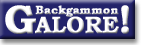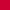TheoryForum Archive : Theory

 Error rates--Repeated ND errors

 From: Joe Russell Address: ez2bblue@aol.com Date: 21 July 2009 Subject: Cube error rates Forum: BGonline.org Forums

```If you are like me you get dinged more than anything else for not doubling
when you should.  Often I will miss a cube by .05 or so for several shakes
in a row. That has led me to consider the fairness of the grading of these
errors.

Say, hypothetically, you had a position that was static for 25 rolls and
for each roll you made a .05 error by not doubling.  Your cumulative error
would be 1.25, but you would have only been .05 better off if you had
doubled at any time.

Now say, in another 25-roll static position, it was wrong to double by .05
and you doubled on the first roll.  Your cumulative error is only .05,
twenty five times less than the other position, but the true cost of the
errors was identical.

I realize in one situation you made one bad decision and in the other you
made 25 bad decisions, but the cost in MWC of the 25 was truly no more than
the cost of the one.
```

 Neil Robins  writes: ```A genuine repeating error can occur when both you and the opponent have a checker on the bar against say four point boards. The problem is that if its a 0.05 missed double, the 0.05 is only valid on the assumption that if the position does repeat you then double. So you need to either take the 0.05 error each time it comes up, or else work out what the initial error would be assuming you repeat the error each time, which is clearly a lot higher than 0.05. ```

 Matt Reklaitis  writes: ```A bot's evaluation represents values related to its own play. So when calculating the size of any individual error, inherent to that calculation is that your future play is similar to the bots. The more your play differs from the bot's, the more the model breaks down. Another way of saying this is that I suspect a bot calculates low error rates more accurately than high error rates. As an example: Lets say a bot thinks a position if worth 73% and is a cube but you think it is worth 72% and is just short of a cube. The bot dings you .05 for a missed double. If you keep repeating the same mistake in sequence, are you really losing .05 each time? Arguably no, because the way you are playing it (assuming the bot's play is correct), you are not really getting 73% out of the position, so you're not really giving up .05. But the argument seems a non-starter because a bot cannot model how a particular human plays -- all it has for error calculation are methods based on its own play. So even if you are arguably giving up less than .05 * N, there's no practical way for a bot to measure that. ```

 Matt Cohn-Geier  writes: ```13 14 15 16 17 18 19 20 21 22 23 24 +---+---+---+---+---+---+---+---+---+---+---+---+---+ | O O O | | X O O O O O | | O | | O O O O | | | | | | | X | | | | | | | | | | | | | | | | | | | | O | | | | | | | X | | X X X X | +---+ | X X X | | O X X X X X | | 2 | +---+---+---+---+---+---+---+---+---+---+---+---+---+ +---+ 12 11 10 9 8 7 6 5 4 3 2 1 X to roll. Let's say the proper cube action here is double/take and your error here in not doubling is .05. This assumes that after fan/fan you will cube. But after fan/fan you won't cube ... so your error is compounded more than .05 would indicate. So regarding the error as just .05 isn't sufficient. ```

 Joe Russell  writes: ```If you take the position and call it a 5-point match, the error in MWC for not doubling is about .9%. If each side dances three times and now you roll without doubling again, you have cost yourself .9% in match winning chances by never doubling, not 2.7%, the cumulative MWC error. In an absurd extension of this, suppose each side danced a 100 times. Do you think that you would have cost yourself 90% MWC, or closer to (and I think exactly) .9% by never doubling? ```

 Matt Reklaitis  writes: ```In the dance 100 times case, you cost yourself 90% and the dice gave you back 89.1%; i.e., you gain .9% from the sequence fan/fan. (Well, here, its not all dice. Opp is giving some back by not doubling too.) ```

 Maik Stiebler  writes: ```I prepared a puzzle involving a discrete random walk to post at some point in this thread: Players A and B play a game of StaticishRace. A game consists of tossing a fair coin and changing the score by +1 or -1 respectively based on the result of the coin toss. The starting score is 0. Player A wins one point and the game ends when the score reaches 50. Player B wins one point and the game ends when the score reaches -50. Before each coin toss, Player A (only player A!, to keep things simple) is given the opportunity to double the stakes, after which Player B can either take or drop (ending the game and losing a single point). A double is allowed only once in a game. 1. What is the optimal strategy for both players? 2. What is the theoretical (assuming optimal play from both sides) equity of the starting position? 3. Assume Player A deviates from optimal strategy by doubling if and only if the current score is +30. What is the practical equity of the starting position then? 4. How much does Player A's deviation from perfect play cost him on average per game? Now put yourself in the position of a bot that knows the optimal strategy and observes the game, not knowing Player A's complete strategy, but noting the wrong plays that follow from the strategy. 5. a) At which points in the game does Player A, following the non-optimal strategy, blunder away theoretical equity by making a wrong play? b) How much equity does each of these wrong plays lose? 6. How often will the opportunity for Player A to make a wrong (equity losing) play arise in a game? Compute both an average value (a) and a distribution (b). 7. Verify that the average number of blunder opportunities (6a) times the cost of a blunder (5b) equals the total cost of Player A's misguided strategy (4). ```

 Bob Koca  writes: ```> 1. What is the optimal strategy for both players? Player A doubles at +25 at which point B has an optional take/pass. That is because B is then has the required 1/4 win chance. A does not double before then as there is no market loss. > 2. What is the theoretical (assuming optimal play from both sides) equity > of the starting position? A has +1/3 equity. Going from -50 to +25 is 75 and A starts 2/3 of the way there. 2/3 - 1/3 = 1/3. > 3. Assume Player A deviates from optimal strategy by doubling if and only > if the current score is +30. What is the practical equity of the > starting position then? A wins 50/80 of the games for an equity of +1/4 > 4. How much does Player A's deviation from perfect play cost him on > average per game? 1/3 - 1/4 = 1/12 Now put yourself in the position of a bot that knows the optimal strategy and observes the game, not knowing Player A's complete strategy, but noting the wrong plays that follow from the strategy. > 5. a) At which points in the game does Player A, following the non- > optimal strategy, blunder away theoretical equity by making a wrong > play? > b) How much equity does each of these wrong plays lose? Only when the game is at exactly 25 does A lose equity. Values below +25 are a no-double and values above +25 are an optional double. The cash is not lost in the next sequence. At +25, B has an optional take/pass. Let's suppose he would pass. Then the equity lost by not doubling at +25 is 1/2 the equity lost by the game reaching +24 (if it reaches +26 it is a cash anyways). At +24, A's theoretical equity is 74/75 - 1/75 = 73/75, a loss of 2/75. So failing to double at +25 costs 1/75 equity. > 6. How often will the opportunity for Player A to make a wrong (equity > losing) play arise in a game? Compute both an average value (a) and a > distribution (b). It happens at least once in 2/3rds of the games. Suppose that the game is exactly +25. We need to find the probability of that state occurring again. This is harder than the previous questions. When you are at +25 you go to +26 half the time. From there you go to 25 before 30 with probability 4/5. The other half the time you go to 24 and then the chance of returning to 25 before -50 is 74/75. (1/2)(4/5) + (1/2)(74/75) = 67/75 chance of a repeat visit to 25 if start from 25. The expected number of times needed to not repeat has a geometric distribution with p = 8/75 and has an expected value of 1/(8/75) = 75/8. The expected total number of visits is thus (2/3) (75/8) = 25/4. The distribution is as follows: Exactly 0 visits occurs with probability 1/3 Exactly 1 visit occurs with probability (2/3)(8/75) Exactly 2 visits occurs with probability (2/3)(67/75)(8/75) Exactly 3 visits occurs with probability (2/3)(67/75)**2(8/75) ... Exactly n visits occurs with probability (2/3)(67/75)**(n-1)(8/75) (This was jointly done with Chris Yep). > 7. Verify that the average number of blunder opportunities (6a) times the > cost of a blunder (5b) equals the total cost of Player A's misguided > strategy (4). (25/4)(1/75) = 1/12. ```

### Theory

Derivation of drop points  (Michael J. Zehr, Apr 1998)
Double/take/drop rates  (Gary Wong, June 1999)
Drop rate on initial doubles  (Gary Wong, July 1998)
Error rate--Why count forced moves?  (Ian Shaw+, Apr 2009)
Error rates--Repeated ND errors  (Joe Russell+, July 2009)
Inconsistencies in how EMG equity is calculated  (Jeremy Bagai, Nov 2007)Janowski's formulas  (Joern Thyssen+, Aug 2000)
Janowski's formulas  (Stig Eide, Sept 1999)
Jump Model for money game cube decisions  (Mark Higgins+, Mar 2012)
Number of distinct positions  (Walter Trice, June 1997)
Number of no-contact positions  (Darse Billings+, Mar 2004)
Optimal strategy?  (Gary Wong, July 1998)
Proof that backgammon terminates  (Robert Koca+, May 1994)Solvability of backgammon  (Gary Wong, June 1998)
Undefined equity  (Paul Tanenbaum+, Aug 1997)Under-doubling dice  (Bill Taylor, Dec 1997)Variance reduction  (Oliver Riordan, July 2003)From GammOnLineLong messageRecommended readingRecent addition

 Book Suggestions Books Cheating Chouettes Computer Dice Cube Handling Cube Handling in Races Equipment Etiquette Extreme Gammon Fun and frustration GNU Backgammon History Jellyfish Learning Luck versus Skill Magazines & E-zines Match Archives Match Equities Match Play Match Play at 2-away/2-away Miscellaneous Opening Rolls Pip Counting Play Sites Probability and Statistics Programming Propositions Puzzles Ratings Rollouts Rules Rulings Snowie Software Source Code Strategy--Backgames Strategy--Bearing Off Strategy--Checker play Terminology Theory Tournaments Uncategorized Variations